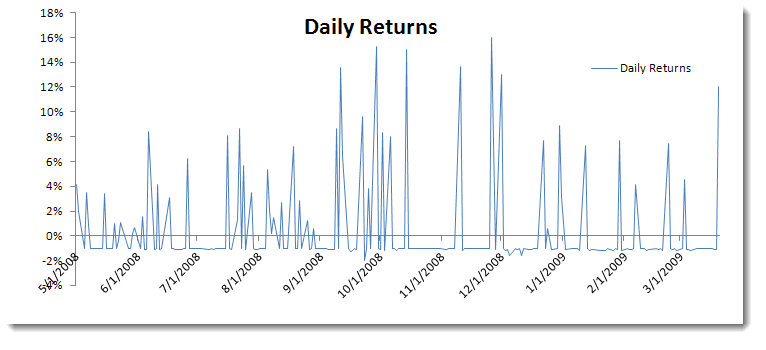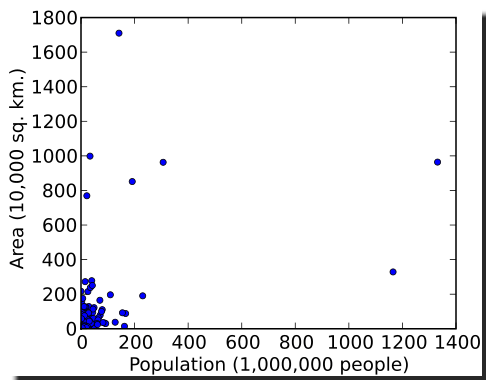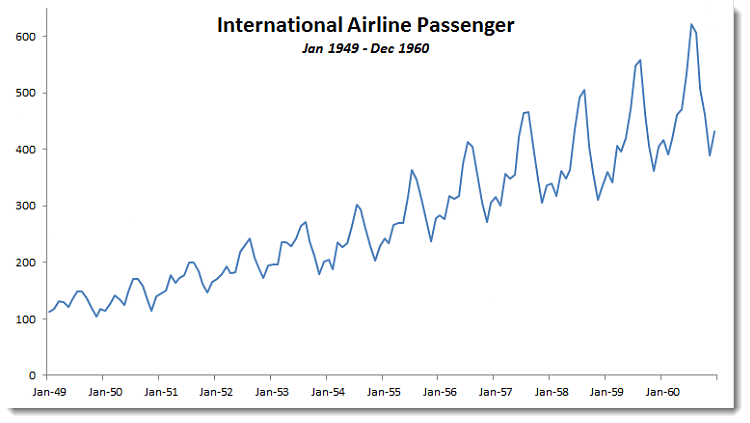# Concentration of Values

In this issue, the fourth tutorial in our data preparation series, we cover data sets for which values are concentrated in a tight range (e.g. proportions), or widely dispersed over several orders of magnitude (e.g. populations, income, rainfall volume, etc.).

For this tutorial, we’ll start by going through a few different value concentration cases: constrained values, mean/variance relationship, etc. Next, we explain their impact on analysis and forecast, and, finally, we present common transformation methods and discuss concerns for mapping results from transformed data scale back to raw data.

## Background

Occasionally, we face a time series sample in which values are naturally restricted to a given range. For instance, mortality rates are restricted between zero and 1, and a trading strategy with a stop‐loss order floors the downside while keeping the upside uncapped; the opposite is the case where a limited sell order is used.Other examples of time series data include the following:

1. Proportions (restricted between 0 and 1, not including the endpoints)
2. Count data (i.e. integers)
3. Positive value
4. Non‐negative values

Furthermore, a data set whose values span several orders of magnitude can prove to be problematic for modeling and forecasting. Examples of such data include Income, population, rainfall volume, etc.## Why do we care?

First, the time series model does not assume any bounds or limits on values that the time series can take, so using those models for a constrained data set may yield poor fitting.

Second, having a floor or a ceiling level in the data set affects the symmetry (or lack of skew) of the values around the mean. This phenomenon can also be difficult to capture using time series models.

Third, a relationship between observation level and local variance may develop and, for the same reasons above, we’ll have to stabilize the variance before doing anything else.## Examining Concentration of Values

In the field of data mining, values concentration is often referred to as “values clustering”; there is a huge volume of literature about grouping, testing, analyzing, etc.

Fortunately, we may be able to get away with a visual examination of the time plot of the data and/or distribution histogram.

Questions:

1. Is the variance changing in relation to the observation levels?
2. Are the data values capped or floor‐leveled?
Please note that the actual level may not be precise enough to allow for potential slippage.
3. Does the distribution show a skew in either direction?

In the case of variance stabilization, the aim of a variance‐stabilizing transformation is to find a simple function ƒ to apply to values $\left \{ x_t \right \}$in a data set to create new values $y_t=f(x_t)$such that the variability of the values of y is not related to their mean value.

We have a values concentration issue in our data... Now what?

Again, the answer is simple: transform the data to a symmetric homoscedastic distribution.

The difficult question is, (Q1) how do I transform the data?

To make things more interesting, the final estimates we obtain are affected when we use transformed data in our analysis original analysis. For example, a logarithmic transformation is often useful for data that have a positive skew to induce symmetry. If we take the forecast mean on the transformed scale and transform by taking the antilog, we get the median which (in this case) is less than the mean forecast of the raw data.

Q2: How can I construct the forecast (mean) value and confidence interval limits from the transformed forecast data?

Once we transform the data to a symmetric homoscedastic distribution, we construct a confidence interval for the forecast. Next, the confidence interval is transformed back to the original scale using the inverse of the transformation that was applied to the data.

This works beautifully for the interval limits and the median, but care must be taken when we interpret the average of the confidence interval.

## Transformation

There are several transformation algorithms to choose from, but care must be taken to choose the best one to treat the root problem. To pick an optimal algorithm, we need to ask a few questions: (1) are we trying to induce symmetry, (2) are we trying to force a normal‐like distribution, or (3) do we wish to stabilize the variance?

1. Logarithmic transformation

$$y_t=\ln(x_t-a)$$ $$x_t>a$$

The logarithmic transformation is often used to induce symmetry in the data and stabilize the variance A variance‐stabilizing transformation aims to remove a mean/variance relationship so that the variance becomes constant relative to the mean. . It is often favored because its results are easy to interpret.

The confidence interval limits can be transformed back, so that the median remains the same throughout the transformation, while the average is not the same. We’ll need to compute its value separately.

Note: In the Airline passenger problem, we used the log of passenger data to stabilize the variance. When we constructed the confidence interval, the forecast value of the transformed data is, by definition, a Gaussian distribution, so the passenger forecast is log‐normally distributed.

The confidence interval average (or mean) is calculated as follow:

$$X_{t+1}=e^{Y_{t+1}+\frac{\sigma^2}{2}}$$

2. Square root transformation

We use the square root transformation for non-negative valued time series.

The square root (and Anscombe) transformation is often applied to stabilize the mean/variance dependency in Poisson‐type data.

The rationale for this originally sprang from the fact that a dataset $\left \{ x \right \}$ is a realization of different Poisson distributions (i.e. the distributions each have different mean values $\mu$.); because the variance is identical to the mean in a Poisson distribution, the variance varies with the mean. However, for the simple variance‐stabilizing transformation $y_t=\sqrt{x_t}$, the sample variance associated with observation will be nearly constant. Please note that an “Anscombe transform” is basically a special case of the square root transformation: $$y=2\sqrt{x+\frac{3}{8}}$$

3. Logit transformation

If values are naturally restricted to be in the range 0 to 1, not including the end‐points, then a logit transformation may be appropriate. This transformation yields values in the range $(-\infty,\infty)$.

$$Logit(p_t)=\ln\left ( \frac{p}{1-p} \right )$$ $$0<p_t<1$$

4. Multiplicative Inverse (reciprocal) transformation

$$y=\frac{1}{x_t}$$

Where $x_t\neq0$

The multiplicative inverse function is probably the simplest transform function as it is self‐inverse.

5. Power (Box‐Cox) Transformation

The Power and, especially Box‐Cox transformations, are often used in time series analysis to transform the data to induce symmetry and resemble a normal distribution.

$$y^{(\lambda )}=\left\{\begin{matrix} \frac{y^\lambda-1 }{\lambda} & \lambda\neq0\\ \ln(y) & \lambda=0 \end{matrix}\right.$$

Note: The logarithmic transform is a special case of a Box‐Cox transformation. The only constraint is $y_t>0$, so for data series with negative values, we can add a constant $\alpha$ such that $y_t+\alpha>0$

$$y^{(\lambda )}=\left\{\begin{matrix} \frac{(y+\alpha)^\lambda-1 }{\lambda} & \lambda\neq0\\ \ln(y+\alpha) & \lambda=0 \end{matrix}\right.$$

The optimal parameters: $\lambda$ and $\alpha$ can be selected by maximizing the log‐likelihood function (LLF) of the transformed data (assuming a Gaussian distribution).

Note that it is not always necessary or desirable to transform a data set to resemble a normal distribution. However, if symmetry or normality are desired, they can often be induced through one of these power transformations.